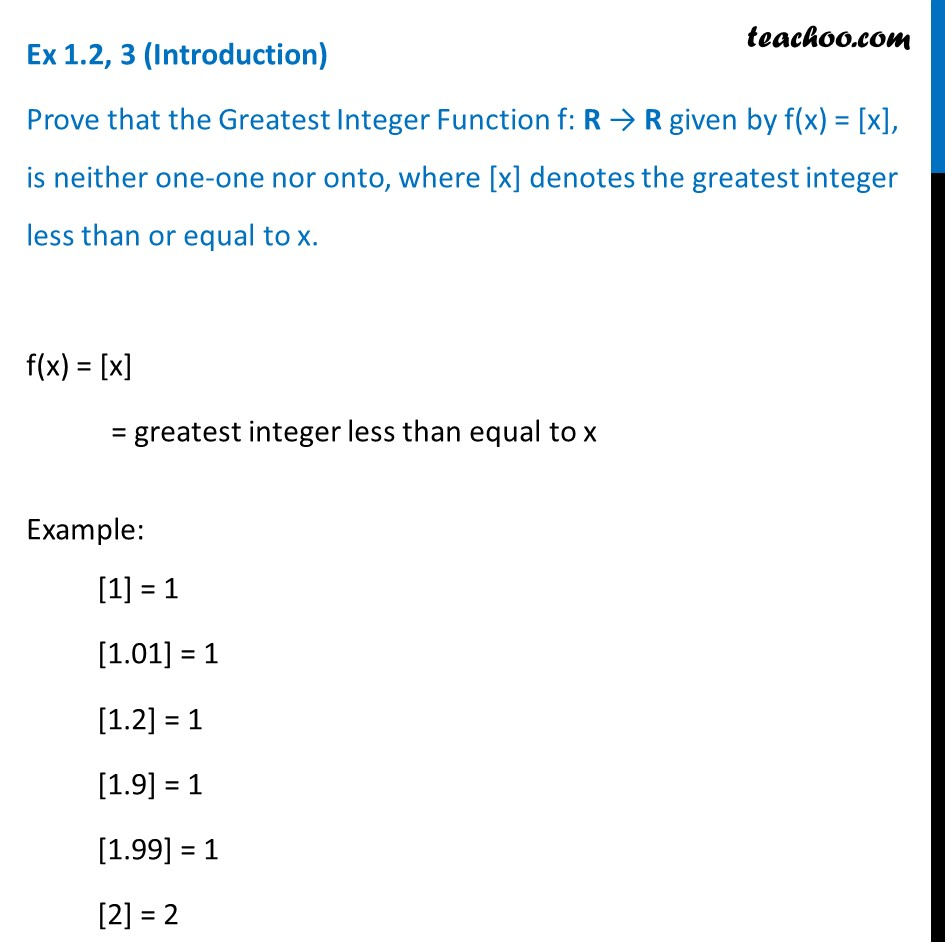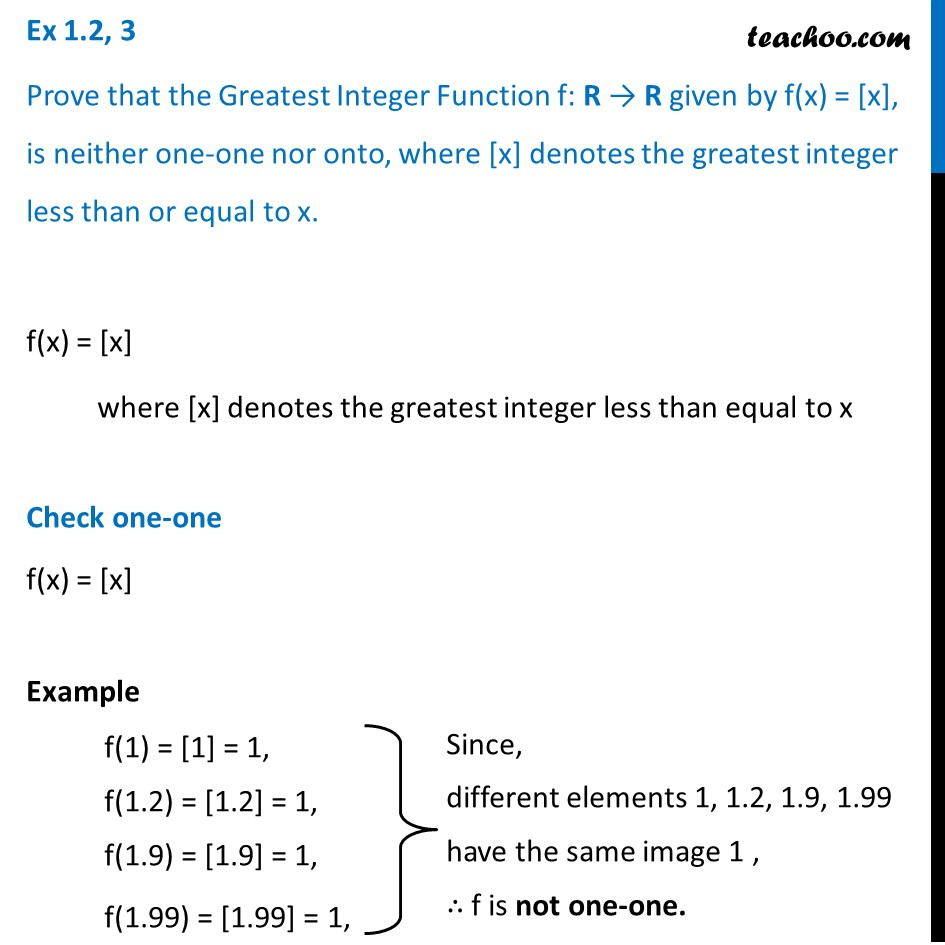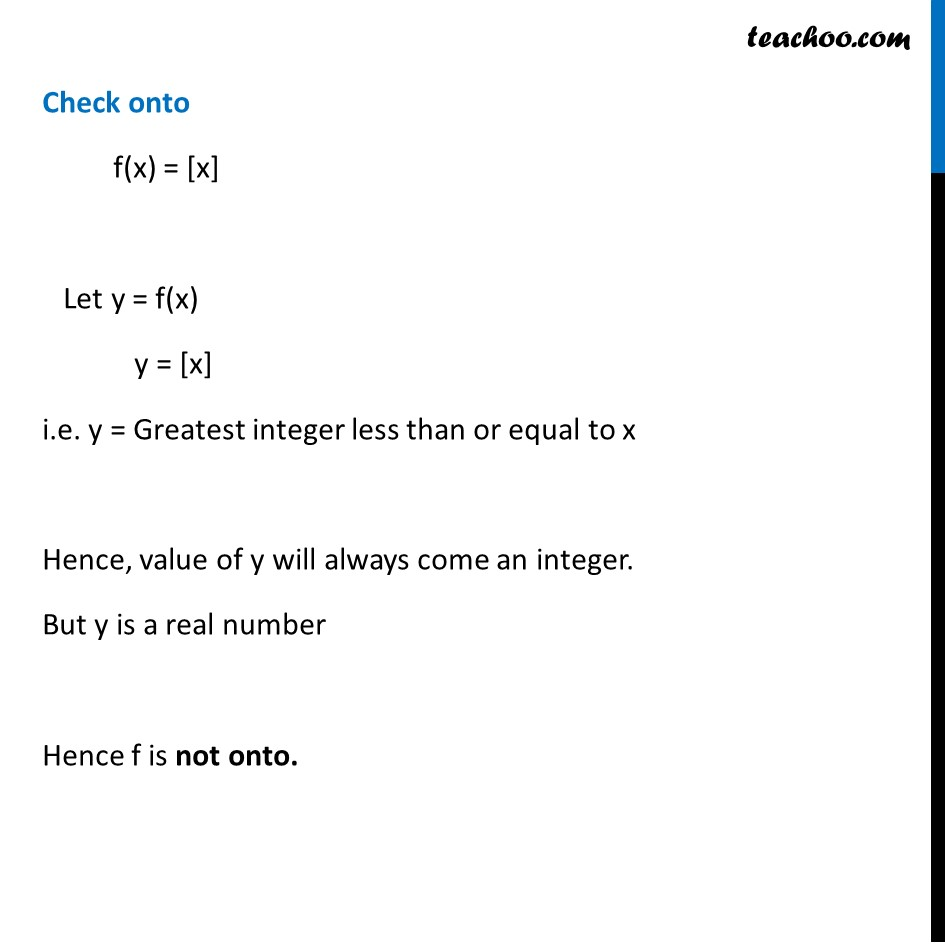Ex 1.2

Chapter 1 Class 12 Relation and Functions
Serial order wiseLearn in your speed, with individual attention - Teachoo Maths 1-on-1 Class

### Transcript

Ex 1.2, 3 (Introduction) Prove that the Greatest Integer Function f: R → R given by f(x) = [x], is neither one-one nor onto, where [x] denotes the greatest integer less than or equal to x. f(x) = [x] = greatest integer less than equal to x Example:  = 1 [1.01] = 1 [1.2] = 1 [1.9] = 1 [1.99] = 1  = 2 Ex 1.2, 3 Prove that the Greatest Integer Function f: R → R given by f(x) = [x], is neither one-one nor onto, where [x] denotes the greatest integer less than or equal to x. f(x) = [x] where [x] denotes the greatest integer less than equal to x Check one-one f(x) = [x] Example f(1) =  = 1, f(1.2) = [1.2] = 1, f(1.9) = [1.9] = 1, f(1.99) = [1.99] = 1, Since, different elements 1, 1.2, 1.9, 1.99 have the same image 1 , ∴ f is not one-one. Check onto f(x) = [x] Let y = f(x) y = [x] i.e. y = Greatest integer less than or equal to x Hence, value of y will always come an integer. But y is a real number Hence f is not onto.